top of page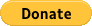## Sponsor a Child in the Village of Tozia, Haiti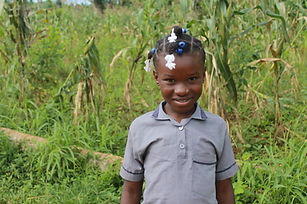I'm E.L.

My number is TOZ011.

I'm J.C.

My number is TOZ022.

I'm P.C.

My number is TOZ025.I'm S.B.

My number is TOZ033.

I'm D.D.

My number is TOZ043.

I'm G.M.C.

My number is TOZ056.I'm G.L.

My number is TOZ056.

I'm R.L.

My number is TOZ063.

I'm R.N.

My number is TOZ071.I'm R.C.

My number is TOZ073.

I'm B.R.P.

My number is TOZ074.

I'm D.S.

My number is TOZ072.I'm L.P.

My number is TOZ075.

I'm R.A.

My number is TOZ076.

I'm R.P.

My number is TOZ077.I'm W.A.

My number is TOZ078.

I'm V.C.

My number is TOZ079.

I'm R.M.

My number is TOZ080.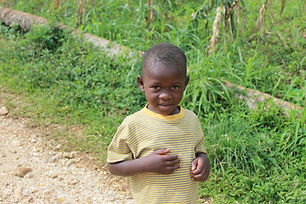I'm E.P.

My number is TOZ081.

I'm T.L.

My number is TOZ083.

I'm V.P.

My number is TOZ082.I'm L.J.B.

My number is TOZ084.

I'm D.S.C.

My number is TOZ086.

I'm D.C.

My number is TOZ085.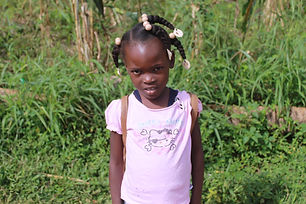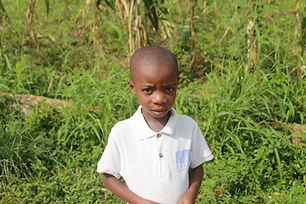I'm L.J.B.

My number is TOZ087.

I'm L.J.B.

My number is TOZ088.

I'm R.J.B.

My number is TOZ089.

bottom of page